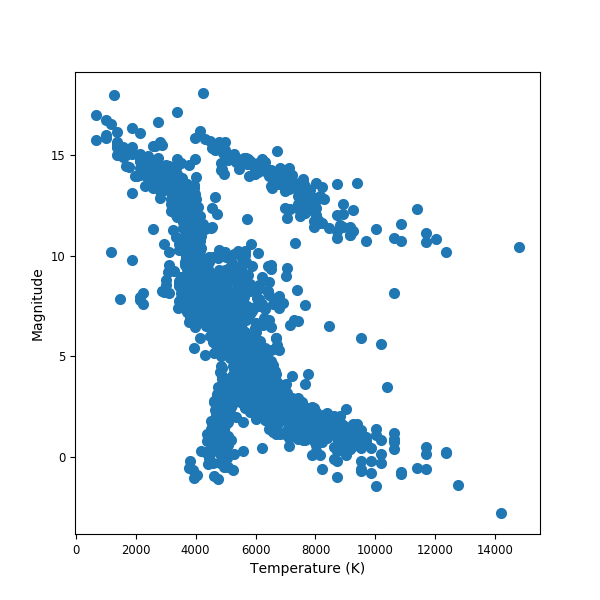# Plotting in Julia (using Python)

Date: 2018-08-12
Last Updated: 2018-08-12

The first step is to have Julia installed as well as a plotting library. I chose to use PyPlot as it uses Matplotlib which I am used to from Python. Install PyPlot using Pkg.add("PyPlot") at the Julia prompt.

Below is an example to generate a Hertzsprung–Russell diagram.

#   Reading in some data.
temperature = data[:,1]
magnitude = data[:,2]

#   Call the plotting library as below

using PyPlot
figure(1,figsize=(6,6))
rc("font", family="sans")
rc("xtick", labelsize="small")
rc("ytick", labelsize="small")

scatter(temperature,magnitude,linewidth=2)

#ylim(0,0.6)
xlabel("Temperature (K)")
ylabel("Magnitude")
#legend(loc="upper left")
savefig("hrdiagram.png")
show()